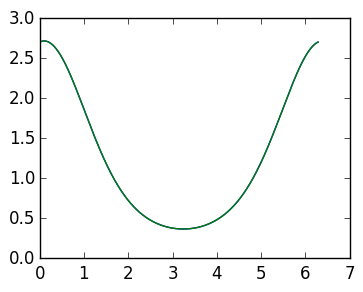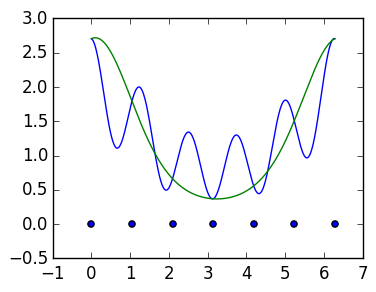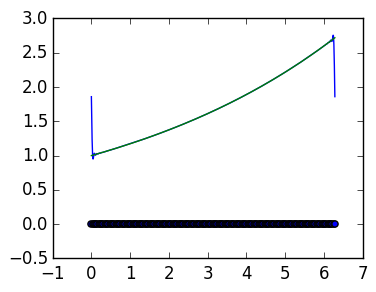# The Discrete Fourier Expansion¶

In this lecture, we will explore the expansion of a function into an approximate Fourier series. That is, suppose we have a periodic function

$$f(\theta) =\sum_{k=-\infty}^\infty \hat f_k e^{i k \theta}$$

for

$$\hat f_k \triangleq {1 \over 2 \pi} \int_0^{2\pi} f(\theta) e^{-i k \theta} d \theta,$$

where we assume the coefficients $\hat f_k$ decay sufficiently fast, so that

$$\sum_{k=-\infty}^\infty |\hat f_k|.$$

(It's beyond the scope of this course, but this condition guarantees that the sum converges to $f$.) We will approximate the function by a finite-dimensional expansion

$$f(\theta) \approx \sum_{k=\alpha}^\beta \hat f_k^n e^{ik \theta}$$

where the approximate coefficients are calculated using the Trapezium rule:

$$\hat f_k^n = {1 \over 2 \pi} Q_n[f(\theta)e^{-ik \theta}]$$

for

$$Q_n[f] = \sum_{j=1}^n f(\theta_j).$$

## Why not quadgk for calculating Fourier coefficients?¶

Why the Trapezium rule? We will see this is not an arbitrary choice: the Trapezium has important properties that lead to the robustness and speed of the approximation. To emphasize this point, let's consider an alternative: just use quadgk. The following sets up a function f̂gk(k) where each coefficient of f is approximated by quadgk:

In :
f=θ->exp(cos(θ-0.1))


Out:
(anonymous function)

We can then evaluate the sum

$$\sum_{k=-8}^8 \tilde f_k e^{ik\theta}:$$

where $\tilde f_k$ is calculated using f̂gk:

In :
ret=0.

for k=-8:8
ret += f̂gk(k)*exp(im*k*0.1)
end

ret-f(0.1)

Out:
-1.1613424000245232e-8 + 0.0im

Unforunately, this very quickly breaks down, requiring an inexorbitant amount of time to evaluate:

In :
@time f̂gk(-9.)

  8.623647 seconds (130.00 M allocations: 2.901 GB, 25.49% gc time)

Out:
3.4302835642957293e-9 + 4.3226999974636545e-9im

## Trapezium rule for calculating Fourier coefficients¶

Instead of quadgk, we use the Trapezium rule:

In :
function trap(f::Function,a,b,n)
h=(b-a)/n
x=linspace(a,b,n+1)

v=f(x)
h/2*v+sum(v[2:end-1])*h+h/2*v[end]
end

trap(f::Function,n) = trap(f,0,2π,n)

Out:
trap (generic function with 3 methods)

The following creates a function f̂(k,n) that returns the Trapezium rule approximation to the $k$th Fourier coefficient, $\hat f_k^n$.

In :
f̂=(k,n)->trap(θ->f(θ).*exp(-im*k*θ),n)/(2π)

Out:
(anonymous function)

We see for sufficiently large $n$, we recover the coefficients accurately:

In :
f̂(-1,20)-f̂gk(-1)

Out:
0.0 + 6.938893903907228e-17im

But this quickly scales up to to large $n$, for approximating

$$f(\theta) \approx \sum_{k=-\beta}^\beta \hat f_k^n e^{i k \theta}.$$
In :
β=100

n=2β+1

ret=0.

for k=-β:β
ret += f̂(k,n)*exp(im*k*0.1)
end

ret-f(0.1)

Out:
4.707345624410664e-14 - 1.1832913578315177e-30im

We will create a routine called dft that returns the approximate Fourier coefficients

$$[\hat f_\alpha^n,\hat f_{\alpha+1}^n,\ldots,\hat f_\beta^n]^\top$$
In :
function dft(f,α,β)
n=β-α+1
Complex128[
trap(θ->f(θ).*exp(-im*k*θ),0.,2π,n)/(2π)   for k=α:β
]
end

Out:
dft (generic function with 1 method)

We also create a routine that allows us to easily evaluate an approximate Fourier series, where fc is a Vector containing

$$[\hat f_\alpha^n,\hat f_{\alpha+1}^n,\ldots,\hat f_\beta^n]^\top$$
In :
function fours(fc::Vector,α,β,θ)
ret=0.+0.im

for k=α:β
ret += fc[k-α+1]*exp(im*k*θ)
end
ret
end

Out:
fours (generic function with 1 method)

Thus we have the approximation:

In :
fc=dft(f,-10,10)
fours(fc,-10,10,0.1)-f(0.1)

Out:
-3.921707403264918e-11 + 0.0im

We can plot this approximate Fourier series:

In :
α,β=-10,10
fc=dft(f,α,β)
using PyPlot

g=linspace(0.,2π,1000)

plot(g,real(map(θ->fours(fc,α,β,θ),g)))
plot(g,f(g));# Aliasing¶

Aliasing is the observation that the discrete Fourier expansion interpolates the true function even when the $\alpha$ and $\beta$ are not chosen to resolve the function. Here is an extreme example: let's take only positive terms:

$$f(\theta) \approx \sum_{k=0}^{n-1} \hat f_k^n e^{i k \theta}$$

Since the true function has both negative and positive Fourier coefficients, we cannot expect this to be accurate, as we see below:

In :
α,β=0,5
f=θ->exp(cos(θ-0.1))
n=β-α+1

fc=dft(f,α,β)

g=linspace(0.,2π,1000)

plot(g,real(map(θ->fours(fc,α,β,θ),g)))
plot(g,f(g))

scatter(linspace(0.,2π,n+1),zeros(n+1));However, at each quadrature point $\theta_j$, the blue curve equals the green curve exactly.

# Gibb's Phenomenon¶

Gibb's phenomenon is the observation that when the function is not periodic, the approximate Fourier series overshoots:

In :
α,β=-100,100

f=θ->exp(θ/(2π))

n=β-α+1

fc=dft(f,α,β)

g=linspace(0.,2π,1000)

plot(g,real(map(θ->fours(fc,α,β,θ),g)))
plot(g,f(g))

scatter(linspace(0.,2π,n+1),zeros(n+1));Investigating this further is beyond the scope of the course.